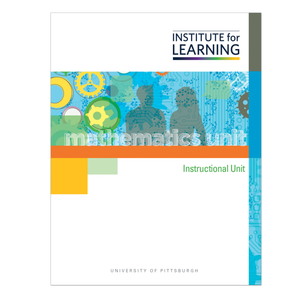Regular price \$149.00 \$0.00 Unit price per

Concepts

• Subtraction with Three-Digit Numbers

Unit includes

• 24 high-level tasks with associated QuickStarts, Practices, Applications, and Quick Writes.
• 3 checkpoints and 6 support activities.
• 1 final performance-based assessment with scoring guide.

Students

• Develop and use flexible strategies for solving addition situations and algorithms, including
• Decomposing by place value and then recomposing the partial sums to arrive at the total sum and
• Rounding to make a friendly number and then compensating for the additional amounts added in/taken away.
• Develop and use flexible strategies for solving subtraction situations and algorithms, including
• Counting back tens and ones or by subtracting like place-value amounts,
• Using addition with an unknown addend as a means for solving subtraction equations, and
• Creating friendly numbers that involve decomposing the subtrahend into smaller portions that can be easily subtracted.